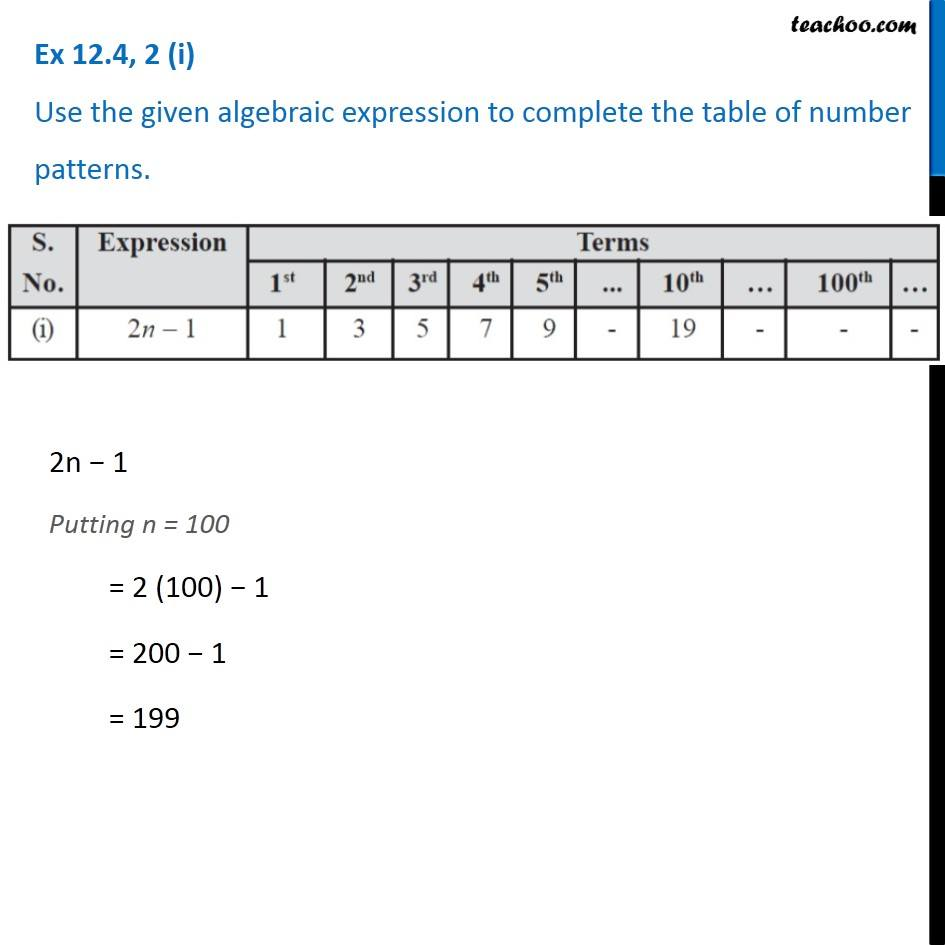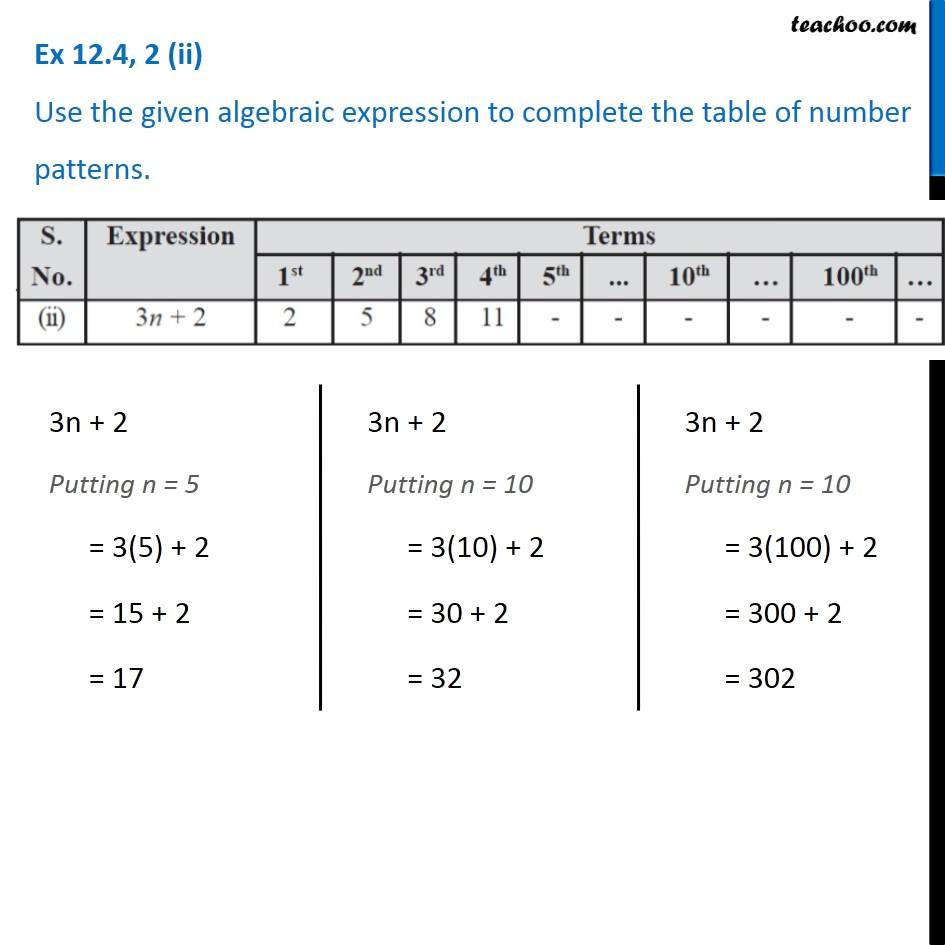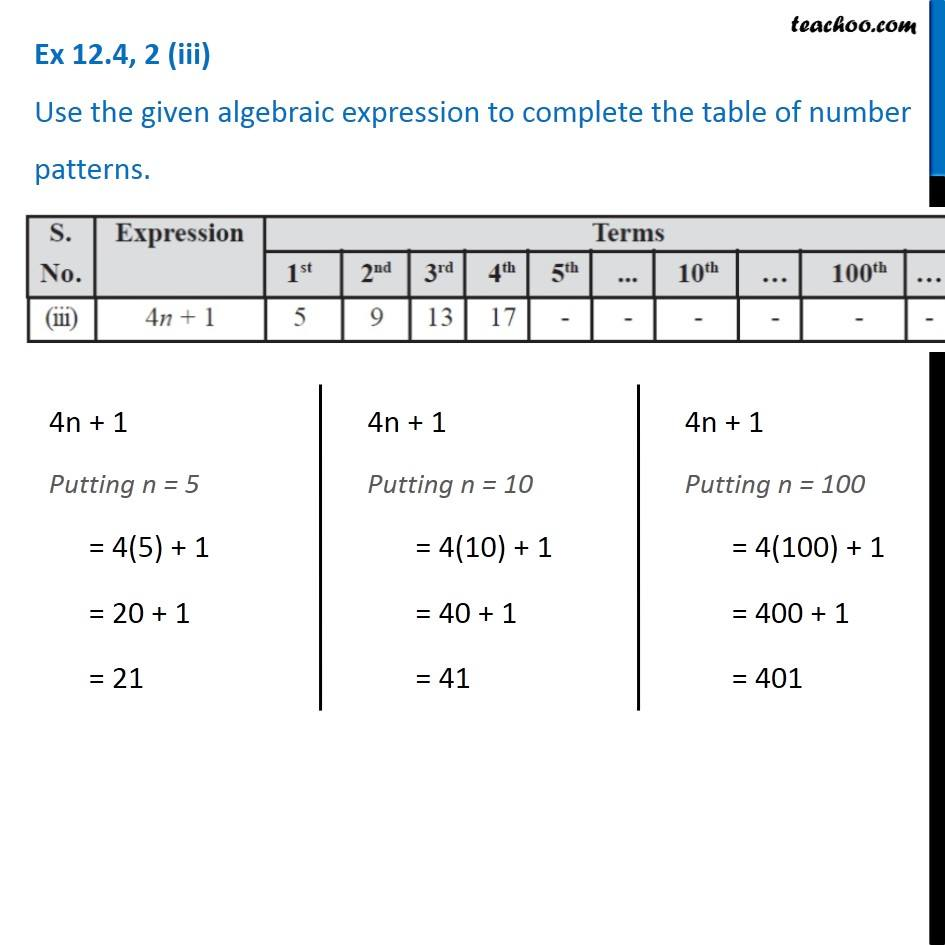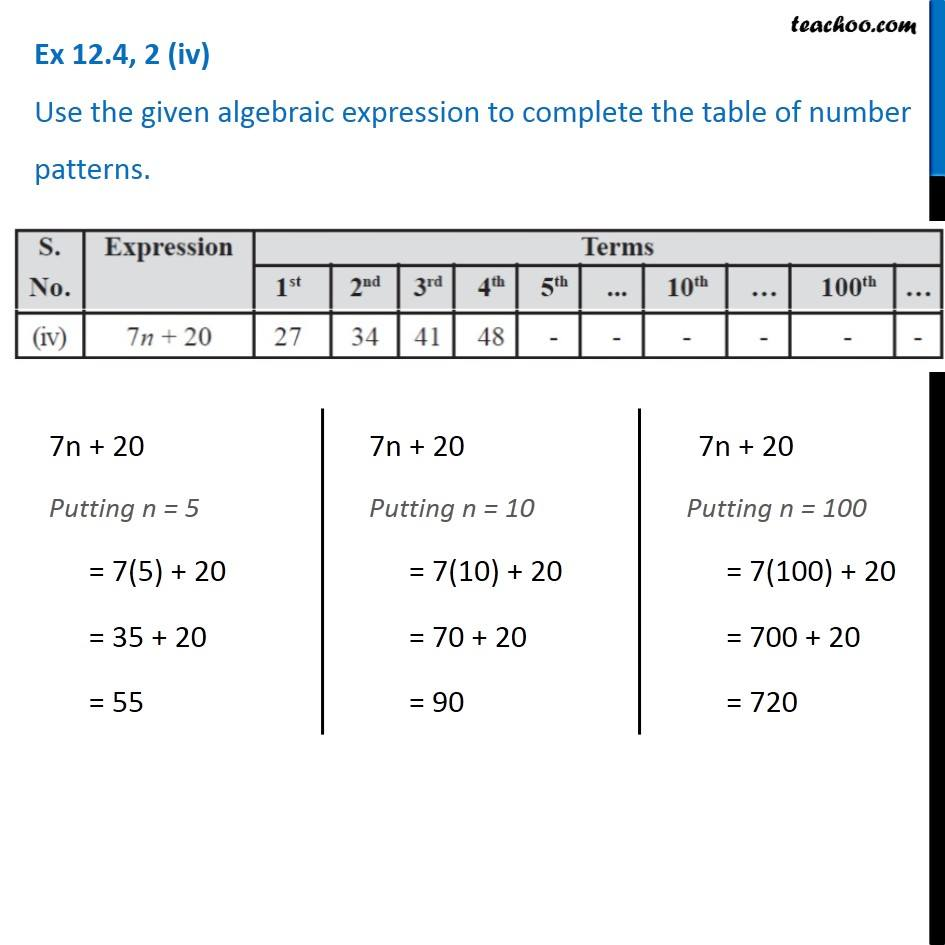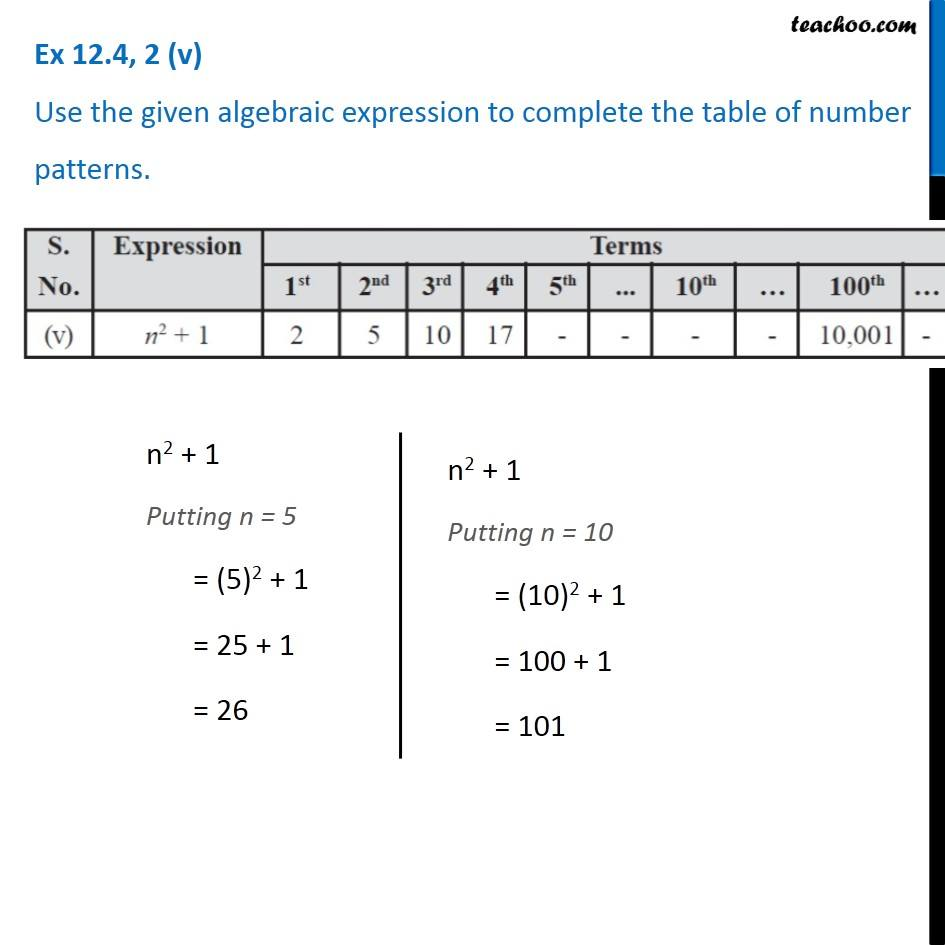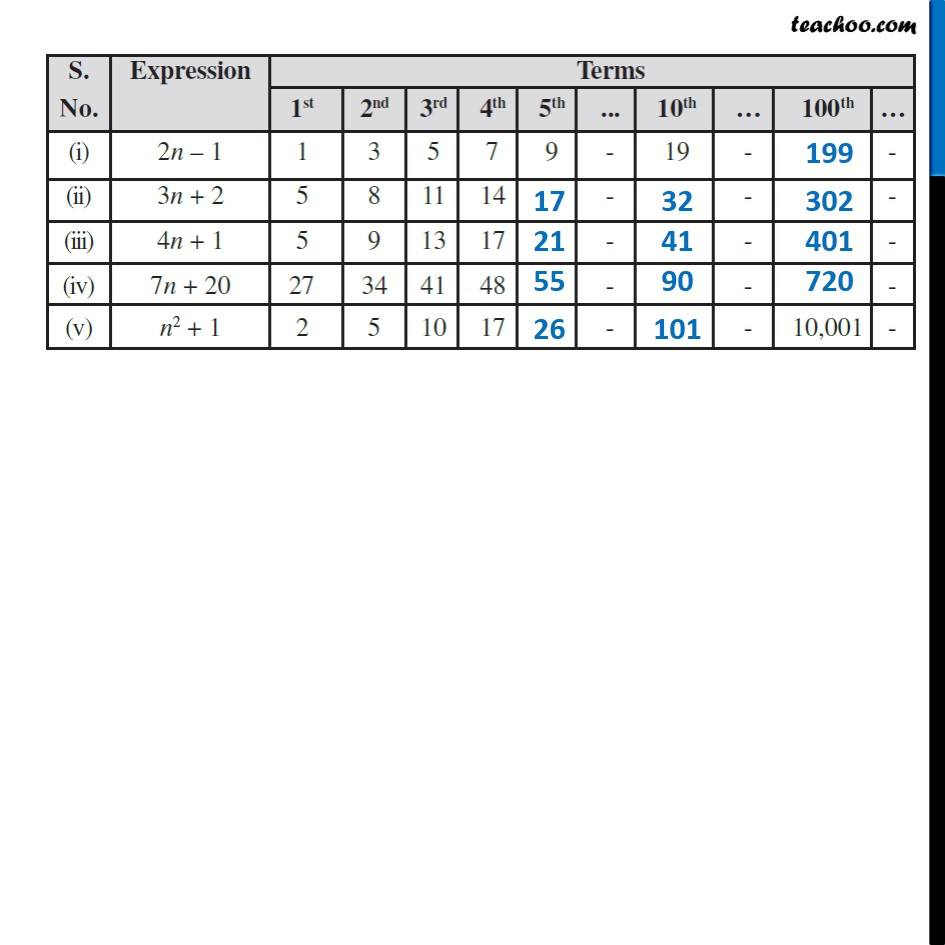Subscribe to our Youtube Channel - https://you.tube/teachoo

1. Chapter 12 Class 7 Algebraic Expressions
2. Serial order wise
3. Ex 12.4

Transcript

Ex 12.4, 2 (i) Use the given algebraic expression to complete the table of number patterns. 2n − 1 Putting n = 100 = 2 (100) − 1 = 200 − 1 = 199 Ex 12.4, 2 (ii) Use the given algebraic expression to complete the table of number patterns. 3n + 2 Putting n = 5 = 3(5) + 2 = 15 + 2 = 17 3n + 2 Putting n = 10 = 3(10) + 2 = 30 + 2 = 32 3n + 2 Putting n = 10 = 3(100) + 2 = 300 + 2 = 302 Ex 12.4, 2 (iii) Use the given algebraic expression to complete the table of number patterns. 4n + 1 Putting n = 5 = 4(5) + 1 = 20 + 1 = 21 4n + 1 Putting n = 10 = 4(10) + 1 = 40 + 1 = 41 4n + 1 Putting n = 100 = 4(100) + 1 = 400 + 1 = 401 Ex 12.4, 2 (iv) Use the given algebraic expression to complete the table of number patterns. 7n + 20 Putting n = 5 = 7(5) + 20 = 35 + 20 = 55 7n + 20 Putting n = 10 = 7(10) + 20 = 70 + 20 = 90 7n + 20 Putting n = 100 = 7(100) + 20 = 700 + 20 = 720 Ex 12.4, 2 (v) Use the given algebraic expression to complete the table of number patterns. n2 + 1 Putting n = 5 = (5)2 + 1 = 25 + 1 = 26 n2 + 1 Putting n = 10 = (10)2 + 1 = 100 + 1 = 101

Ex 12.4